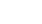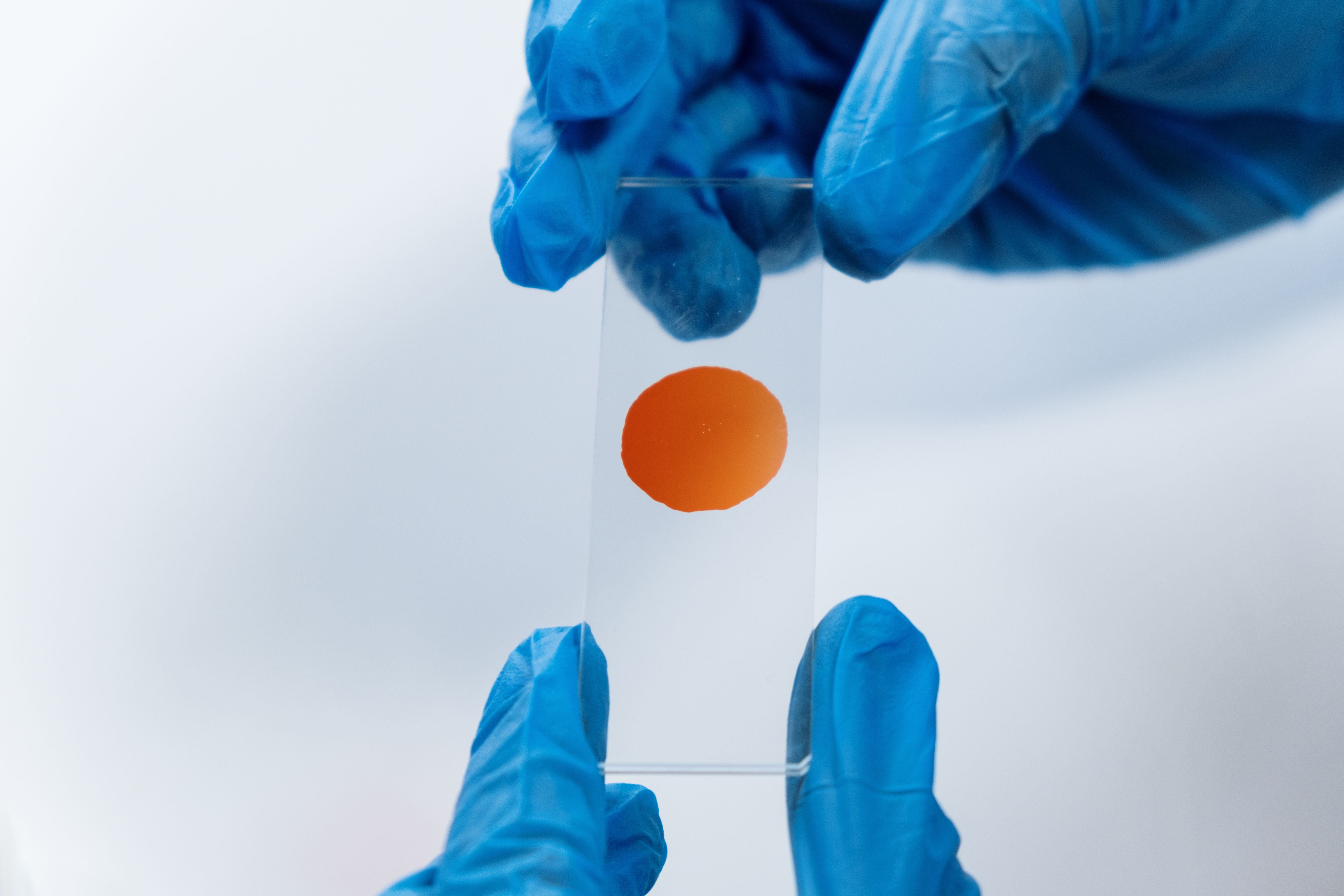# Shaking off the Rust### Febuary 12th, 2022Difficulty: Intermediate

Shaking off the Rust is a series of exercises with the Rust programing language. The purpose of the series is to improve both my and my dear reader’s abilities with Rust by building things. Plus, by actually building stuff, we'll learn about an array of technological concepts in the process. In this installment, we’re doing a bit of bioinformatics.

This installment's Github repo: https://github.com/josht-jpg/rust_dna_search

### Biology Primer

Before beginning, you may find a short biology session worthwhile.

Deoxyribonucleic acid (DNA) consists of a chain of sugars and phosphates which string together nitrogenous bases . These bases, called nucleotides, come in four types: adenine, guanine, cytosine, and thymine, often abbreviated to A, G, C, and T. Genetic information is encoded by the sequence of nucleotides in DNA .

A set of three nucleotides is a codon. Codons specify amino acids, which are the building blocks of proteins .

In computer programs, we can represent a DNA molecule as a string of nucleotides (here I’m using string in the computational sense). This makes the analysis of DNA particularly well suited to the area of algorithms and computation.

### Getting Started

We’ll begin by creating a new library with Cargo.

cargo new dna_search --lib
cd dna_search

### Declaring some Types

A great way to represent a nucleotide in our Rust program is with an enum:

//  lib.rs

#[derive(Copy, Clone, PartialEq, Eq, PartialOrd, Ord, Hash)]
enum Nucleotide {
A,
C,
G,
T,
}

If the derive attribute is new to you, I recommend taking a glance at Appendix C of The Rust Programming Language Book. As we go through the code, I’ll explain when and why we need each of these traits.

To prevent contaminating our code with magic literals, I’ve created a constant for the number of nucleotides in a codon:

const NUCLEOTIDES_IN_CODON: usize = 3;

We’ll declare types for a codon and a gene. A codon can be represented as a tuple of three nucleotides, and a gene can be represented as a vector of codons.

type Codon = (Nucleotide, Nucleotide, Nucleotide);
type Gene = Vec<Codon>;

### Converting a String to Nucleotides

We’re going to assume that the data we are working with is a string of nucleotides, e.g.

let gene_str = "ATTCGCA";. So we’ll want a helper function to convert a string slice into a vector of Nucleotides. We’ll call this str_to_nucleotides.

fn str_to_nucleotides(s: &str) -> Vec<Nucleotide> {
s.chars()
.map(|c| match c {
'A' => Nucleotide::A,
'C' => Nucleotide::C,
'G' => Nucleotide::G,
'T' => Nucleotide::T,
_ => panic!("Not a nucleotide"),
})
.collect()
}

The ability to use abstractions like map without hurting performance is one of the reasons why I love Rust so darn much.

### Nucleotide Frequencies

Let’s warm up with a program to calculate nucleotide frequencies of a piece of DNA. That is, we’ll count the number of occurrences of each nucleotide. Here’s some pseudocode for this algorithm:

nucleotide_frequency(dna) returning hash map with keys of type Nucleotide and values of type integer {
frequencies = empty HashMap;
insert (A, 0) into frequencies
insert (T, 0) into frequencies
insert (G, 0) into frequencies
insert (C, 0) into frequencies

for each nucleotide in dna {
frequencies[nucleotide] = frequenices[nucleotide] + 1
}

return frequencies
}

And here’s the Rust implementation:

use std::collections::HashMap;

fn nucleotide_frequency(dna: &[Nucleotide]) -> HashMap<Nucleotide, u32> {
let mut frequencies: HashMap<Nucleotide, u32> = HashMap::from([
(Nucleotide::A, 0),
(Nucleotide::T, 0),
(Nucleotide::G, 0),
(Nucleotide::C, 0),
]);

for nucleotide in dna {
increment_nucleotide_count(&mut frequencies, nucleotide);
}

return frequencies;
}

fn increment_nucleotide_count(frequencies: &mut HashMap<Nucleotide, u32>, nucleotide: &Nucleotide) {
*frequencies.get_mut(nucleotide).unwrap() += 1;
}

The need arises for our Nucleotide enum to derive the Hash trait. Implementing Hash allows us to use Nucleotides as keys in a HashMap . Without deriving Hash, the compiler will throw this error: the trait bound Nucleotide: Hash is not satisfied.

I’ll also make note of this ugly line of code:

*frequencies.get_mut(nucleotide).unwrap() += 1;

This increments a value within our frequencies HashMap. I’ll go into some gory details. Skip ahead if you aren’t curious.

The Rust docs tell us that the get_mut method takes a possible key and “returns a mutable reference to the value corresponding to the key” .

There is generally no guarantee that a HashMap contains the key given to get_mut. Thus, get_mut returns an Option. If you’re unfamiliar with Options in Rust, I suggest reading this section of the Rust Programming Language Book.

Since get_mut returns an Option, the unwrap method is necessary. The unwrap method accesses the data within the Option’s Some value .

There’s a similar method to unwrap that we could use here - the expect method. expect is like unwrap, but allows us to specify an error message if the Option it is operating on holds None . In most cases, I prefer using the expect method because it makes the code more explicit and helps with debugging. But in this case, we actually know that the Option returned from get_mut won’t be None (since we’ve initialized frequencies with values for each kind of Nucleotide). This makes expect unnecessary.

Finally, note that we cannot preform the += operation on the &mut i32 type. This is why we dereference (in Rust, we deference with *): *frequencies.get_mut(nucleotide).unwrap() += 1

Here’s a test for our nucleotide_frequency function:

// lib.rs

mod tests {
use super::*;

#[test]
fn nucleotide_frequency_test() {
let dna_str = "ATATCTTAGAGGGAG";
let freq = nucleotide_frequency(&str_to_nucleotides(&dna_str));

assert_eq!(freq.get(&Nucleotide::A), Some(&5));
assert_eq!(freq.get(&Nucleotide::T), Some(&4));
assert_eq!(freq.get(&Nucleotide::C), Some(&1));
assert_eq!(freq.get(&Nucleotide::G), Some(&5));
}
}

### Searching DNA for a Codon

Much like the bit is the fundamental unit of computation, the gene, which is a section of DNA, is the fundamental unit of biological information . Searching through a gene for a specific codon is a common problem in bioinformatics . Let’s try it.

We’ll make another helper function, similar to str_to_nucleotides, to convert a string slice representing DNA into a Gene. We’ll call the function str_to_gene:

fn str_to_gene(s: &str) -> Gene {
let nucleotides = str_to_nucleotides(s);
let num_nucleotides_in_codons = nucleotides.len() - (nucleotides.len() % NUCLEOTIDES_IN_CODON);

return (0..num_nucleotides_in_codons)
.step_by(NUCLEOTIDES_IN_CODON)
.map(|i| (nucleotides[i], nucleotides[i + 1], nucleotides[i + 2]))
.collect();
}

We are not considering any nucleotides that are not a part of a codon. For example, if we are given ATTCACGG, the nucleotides GG will be ignored as they do not fit within a multiple of three. This is why num_nucleotides_in_codons exists.

We group the remaining nucleotides into codons with the step_by method, which allows us to take custom sized steps.

The line .map(|i| (nucleotides[i], nucleotides[i + 1], nucleotides[i + 2])) requires implementation of Copy and Clone triats. The Rust docs tell us that Clone provides the ability to explicitly duplicate an object  and that Copy (which requires Clone) provides the ability to duplicate values by copying bits .

If Nucleotide did not implement these traits, the compiler would scold us with:

cannot move out of index of Vec<Nucleotide>

move occurs because value has type Nucleotide, which does not implement the Copy trait

To search the given gene for a codon, we’ll use binary search:

Binary search requires the structure it’s operating on to be sorted . So we’ll start by sorting the codons in our gene by alphabetical order.

We’ll continue the binary search algorithm by comparing our target codon, $c$﻿, with the middle codon in the sorted gene (that is, the ($\frac{n}{2}$﻿)nd codon, where $n$﻿ is the number of codons in the gene).

If $c$﻿ is less* than the gene’s ($\frac{n}{2}$﻿)nd codon, we discard the second half of the sorted gene. If $c$﻿ is greater, we discard the first half.

Binary search works by repeating this process until we either find the target codon or complete $\log_2 n$﻿ steps (if the target codon is not present in the gene) .

*By less, I mean $c$﻿ proceeds the gene’s ($\frac{n}{2}$﻿)nd codon in lexical order.

We’ll create a function called binary_search_for_codon to do all that. Again, I’ll provide pseudocode, followed by a Rust implementation.

binary_search_for_codon(gene, target_codon) returning bool {
sort(gene)

low = 0
high = length(gene) - 1

while low <= high {
middle_codon_index = floor((low + high) / 2)
middle_codon = gene[middle_codon_indedx]

if gene[middle_codon_index] < target_codon {
low = middle_codon_index + 1
} else if gene[middle_codon_index] > target_codon {
high = middle_codon_index - 1
} else {
return true
}
}

return false
}

fn binary_search_for_codon(sorted_gene: &Gene, target_codon: &Codon) -> bool {
let mut low = 0;
let mut high = sorted_gene.len() - 1;

while low <= high {
let middle_codon_index = (low + high) / 2;
let middle_codon = &sorted_gene[middle_codon_index];

if middle_codon < target_codon {
low = middle_codon_index + 1;
} else if middle_codon > target_codon {
high = middle_codon_index - 1;
} else {
return true;
}
}

return false;
}

Here’s a test for binary_search_for_codon:

mod test {

/*...*/

#[test]
fn binary_search() {
let gene_str = "ATATCTTAGAGGGAGGGCTGAGGGTTTGAAGTCC";
let mut gene = str_to_gene(gene_str);
gene.sort();

let ata: Codon = (Nucleotide::A, Nucleotide::T, Nucleotide::A);
let atc: Codon = (Nucleotide::A, Nucleotide::T, Nucleotide::C);
let agg: Codon = (Nucleotide::A, Nucleotide::G, Nucleotide::G);
let tcc: Codon = (Nucleotide::T, Nucleotide::C, Nucleotide::C);

assert!(binary_search_for_codon(&gene, &ata));
assert!(!binary_search_for_codon(&gene, &atc));
assert!(binary_search_for_codon(&gene, &agg));
assert!(!binary_search_for_codon(&gene, &tcc));
}

For gene.sort() to work, Nucleotide needs to implement the Ord trait. Values of a type that implements Ord can be compared relative to one another . To implement Ord, the traits PartialOrd and Eq are required (and Eq requires PartialEq).

### Naive Exact Matching

The exact matching problem consists of finding all occurrences of a pattern string $p$﻿ within a larger text string $t$﻿, where the length of $p$﻿ is necessarily less than the length of $t$﻿. To record the occurrences of $p$﻿ in $t$﻿, we’ll record the offests, which are the leftmost occurrences of $p$﻿ in $t$﻿ .

For example, if we have a pattern 'ATT' and text 'ATTGATTC', the offsets will be 0 and 4.

Optimizations for the exact matching problem can get complex. For now, we’ll only concern ourselves with a naive or brute force solution. The naive solution consists of looking at all possible positions in $t$﻿ where $p$﻿ could occur and checking if there is a match .

Let’s finish this session by using the naive matching algorithm to find the positions of a pattern of nucleotides within a larger strand of DNA. As always, we’ll start with pseudocode:

naive_match(dna, target_sequence) returning list of integers {
target_occurance_at = empty list

possible_starting_positions = 0 to (length(dna) - length(target_sequence) + 1)

for i in possible_starting_possitions {
for j in 0 to length(target_sequence) {
if dna[i + j] != target_sequence[j] {
break
}

if j == length(target_sequence) - 1 {
target_occurance_at.push(i)
}
}
}

return target_occurance_at
}

And now for the Rust implementation:

fn naive_match(dna: &[Nucleotide], target_sequence: &[Nucleotide]) -> Vec<usize> {
assert!(dna.len() >= target_sequence.len());

let mut target_occurance_at = Vec::new();

let possible_starting_positions = 0..(dna.len() - target_sequence.len() + 1);

for i in possible_starting_positions {
for j in 0..target_sequence.len() {
if dna[i + j] != target_sequence[j] {
break;
}

if j == target_sequence.len() - 1 {
target_occurance_at.push(i);
}
}
}

return target_occurance_at;
}

This implementation is pretty straightforward - no tricky Rust maneuvers required.

Let’s write a test to see if that’s working:

mod tests {

/*...*/

#[test]
fn naive_match_test() {
let dna = str_to_nucleotides("ATATCTTAGAGGGAGGGAGG");
let target_sequence = str_to_nucleotides("AGG");

let match_indices = naive_match(&dna, &target_sequence);

assert_eq!(match_indices.len(), 3);
assert_eq!(match_indices, 9);
assert_eq!(match_indices, 13);
assert_eq!(match_indices, 17);
}
}

If that passes for you, then congratulation, today's set of exercises are complete!

Thanks for coding with me, friends. Becoming a great Rust programmer is a marathon - I hope this session has brought you one step closer.

### References

 Rust docs for the Hash Trait

## Support Me

Creating and running Shaking off the Rust is one of the most fulfilling things I do. But it's exhausting. By supporting me, even if it's just a dollar, you'll allow me to put more time into building this series. I really appreciate any support.

The only way to support me right now is by sponsoring me on Github. I'll probably also set up Patreon and Donorbox pages soon.

Thank you so much!